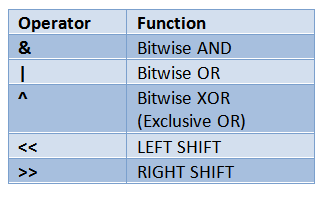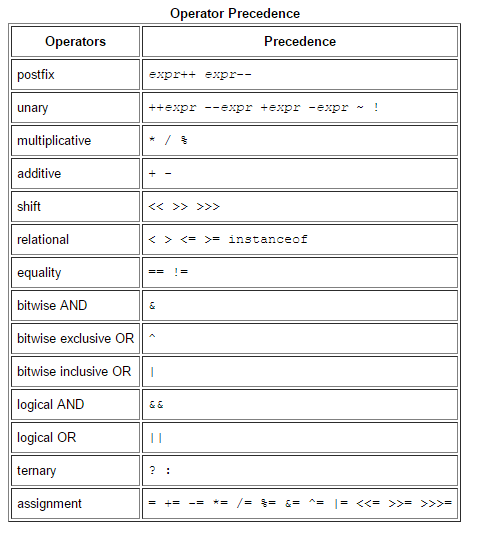# Java | Operators

05 min read

Operators:

1.  Arithmetic Operators:

Same as in C or C++, with the following additional features.

• "%' operator can be applied for floating-point types also. (eg) double y = 42.25;

y % 10 = 2.25

• + + and -- operator can be used for floating-point types also. (eg) float a = 3.5f;

System.out.printIn(++a); —> 4.5

2. Bitwise Operators:The above operators are similar to C.

<< - left shift operator shifts the bits of the given number specified number of times to the left.

(eg) a = a << 2; —> shifts the bits of 'a', 2 times, towards the left.

Additionally, Java provides the following 2 bitwise operators.

>>> shift right zero fill (or) unsigned shift right operator

>>>= unsigned shift right assignment

3. Relational Operators:

== , != , > , < , >= ,  <=

Outcome of these operators is the boolean value (unlike in C or C++, where 'I' or '0' is returned).

(eg) int a = 4, b = 1;

boolean c = a > b;

System.out.println(c);   -> 0/P: true

If any integer variable has to be used in a loop or branching statement, it must be written as int n = 65;

if(n = = 0) (or) if(n != 0)               while(n = = 0) (or) while(n !=0)

It cannot be written as while(n)

{

.......

}

because in java, a positive value does not meanctrue and zero value does not mean false.

4. Boolean Logical Operators:

Operates only on boolean operands.

& -  logical AND

I -  logical OR

^ -  logical XOR

!= -  logical NOT

&= -  AND Assignment

I= - OR Assignment .

^= - XOR Assignment

= =  - Equal to

!=  - Not Equal to

&& - short circuit AND

II - short circuit OR

?: - Ternary Operator

instanceof Operator: It returns true when the object on the left hand side is an instance of the class on the right hand side. It is used to determine whether the object belongs to a particular class or not.

(eg) e instanceof employee will be true if 'e' object belongs to the class 'employee', else false.

Dot Operator:  Used to access the instance variables and methods of class object. (eg) e.enarne , e.salary , e.increment( )

Operator Precedence:

Note: All binary operators except assignment operators follow L --> R associativity.### Relevant exercises

POST A NEW COMMENT

• Input (stdin)

Output (stdout)

Input (stdin)

Your Output (stdout)

Expected Output

Compiler Message

Input (stdin)

`2    3`

Your Output (stdout)

`5`

Expected Output

`5`

Compiler Message

`5`